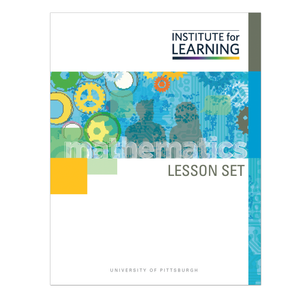# Investigating Rational Exponents (Algebra 2)

Regular price \$6.25 \$0.00 Unit price per

In this lesson set, students investigate the characteristics of expressions and equations with rational exponents. Particular attention is paid to writing equivalent expressions, graphing equations with rational exponents, and comparing numbers with rational exponents.

Through engaging in the lessons in this lesson set, students will:

• deepen understanding of the properties of exponents by examining rational exponents;
• recognize and write expressions using rational exponents in equivalent forms;
• sketch graphs of equations with rational exponents in the first quadrant; and
• compare the relative size of numbers written using rational exponents.

Standards: N-RN.A.1, N-RN.A.2